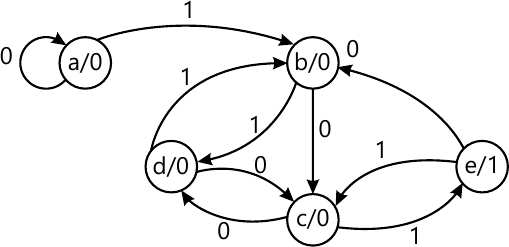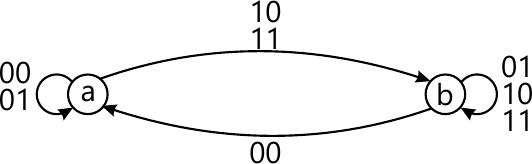MORE IN Analog and Digital Electronics
VTU Computer Science (Semester 3)
Analog and Digital Electronics
June 2013
Total marks: --
Total time: --
INSTRUCTIONS
(1) Assume appropriate data and state your reasons
(2) Marks are given to the right of every question
(3) Draw neat diagrams wherever necessary

1 (a) Write the truth table of the logic circuit having 3 inputs, A, B and C and the output expressed as Y=ABC+ABC. Also simplify the expression using Boolean algebra and implement the logic circuit using NAND gates.
6 M
1 (b) Name universal gates. Realize basic gates using NAND gates.
8 M
1 (c) Explain positive and negative logic.
6 M

2 (a) Give sum-of-product of sum circuit for.
f(A, B, C, D)=∑m(6,8,9,10,11,12,13,14,15)
8 M
2 (b) Find essential prime implicants for the Boolean expression by using Quine-McClunky method.
f(W,X,Y,Z)=∑(1,3,6,7,8,9,10,12,13,14)
12 M

3 (a) Design a 16 to 1 multiplexer using two 8 to 1 multiplexer and one 2-to-1 multiplexer.
6 M
3 (b) Explain n-bit magnitude comparator.
8 M
3 (c) Design 7-segments decoder using PLA.
6 M

4 (a) Explain Schimmit trigger.
6 M
4 (b) Give state transition diagram of SR, D, JK and T FlipFlops.
8 M
4 (c) Show how a D Flip-Flop can be converted into JK-Flip Flop.
6 M

5 (a) Design 3-bit PISO (Use D -FlipFLop).
6 M
5 (b) Design two 4-bit serial adder.
6 M
5 (c) Design 4-bit Johnson counter with state table.
8 M

6 (a) Design Synchronous mod 6 up-counter using JK - Flip Flop.
10 M
6 (b) Explain digital clock with block diagram.
10 M

7 (a) Reduce state transition diagram (Moore model) Fig.Q7(a) given below by,
(i) Row elimination method and
(ii) Implication table method, with partition table.12 M
7 (b) Design an asynchronous sequential logic circuit for state transition diagram shown below Fig.Q7(b).8 M

8 (a) Explain with logic diagram 3-bit simultaneous A/D converters.
10 M
8 (b) Explain with neat diagram, single-slope A/D converter.
10 M

More question papers from Analog and Digital Electronics